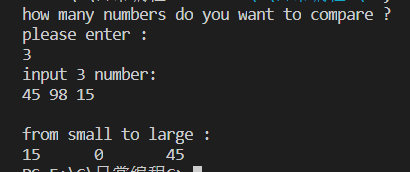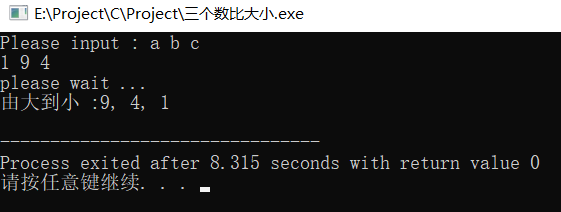• C语言个数比大小
C语言三个数比大小
代码如下：
#include <stdio.h>
int main()
{
int a,b,c;
scanf("%d%d%d",&a,&b,&c);
if(a>b)                  //判断a与b之间的大小关系，如果a大于b，那么就把b中的值赋到a中
{
a = a+b;			 //如果a小于b，那么就不变，总之一定要保证a中是存储着当前最小的值
b = a-b;
a = a-b;
}
if(a>c)                  //同上，判断a与c之间的大小关系，如果c小于a，那么就把c中的值赋到a中
{
a = a+c;             //由第一个if可以得到那时的a中存储的一定是当时两个数的最小的值，把那个值再与c比较，就可得出三个数中的最小值
c = a-c;
a = a-c;
}
if(b>c)                  //判断出最小值了，但最大值和第二大的值没有得出，再进行第三次if判断，判断出最大值
{
b = b+c;             //如果b>c则把b和c中的值互换，如果b<c则不变，一定要保证c中存储的是最大值，最小值和最大值都确定了，那还剩一个值就是中间的值
c = b-c;
b = b-c;
}
printf("%d<%d<%d",a,b,c);//此时a，b，c中的数都是按大小排序的
return 0;
}



展开全文• printf("请输入两个实数：\n"); scanf("%d%d",&a,&b); if(a>b) { printf("%d,%d",b,a); } else printf("%d,%d",a,b); return 0; } //一次比较 ，一次交换，为了实现互换，必须借助于第3个变量。 ...
//两次比较，比较简单。
/*#include <stdio.h>
int main(void)
{
int a,b;
printf("请输入两个实数：\n");
scanf("%d%d",&a,&b);
if(a>b)
{
printf("%d,%d",b,a);
}
else
printf("%d,%d",a,b);
return 0;
}

//一次比较 ，一次交换，为了实现互换，必须借助于第3个变量。

#include<stdio.h>
int main (void)
{
int a,b,t;
printf("请输入两个实数：\n");
scanf("%d%d",&a,&b);
if(a>b)
{
t=a;
a=b;
b=t;
}
printf("%d,%d",a,b);
return 0;
}

//三个数比较大小

#include<stdio.h>
int main (void)
{
int a,b,c,t;
printf("请输入三个实数：\n");
scanf("%d%d%d",&a,&b,&c);
if(a>b)
{
t=a;
a=b;
b=t;
}
if(a>c)
{
t=a;
a=c;
c=t;
}
if(b>c)
{
t=b;
b=c;
c=t;
}
printf("%d,%d,%d",a,b,c);
return 0;

}



展开全文• 注：用C语言编写，运用数组，for嵌套等 #include <stdio.h> int main() { int b, i, j, t; printf("how many numbers do you want to compare ?\n"); printf("please enter : \n"); scanf("%d",&b);...

注：用C语言编写，运用指针，数组，for嵌套等
1、经典的冒泡法

#include <stdio.h>

int main()
{
int b, i, j, t;
printf("how many numbers do you want to compare ?\n");
scanf("%d",&b);
printf("input %d number: \n" ,b);
int a[b];
for(i = 0; i < b; i++) {
scanf("%d",&a[i]);
}
printf("\n");
for(j=0; j < b-1; j++)
for(i=0; i < b-j; i++)
{
if(a[i] > a[i+1])
{
t = a[i];
a[i] = a[i+1];
a[i+1] = t;
}
}
printf("from small to large : \n");
for(i = 0; i < b; i++)
printf("%d\t", a[i]);
printf("\n");

return 0;
}


这段代码本想用来比较多个数比大小
其实我本想比较无数个数
可是程序似乎哪里出错了（有可能是数组那里出错了）
比如在想比较3个数的大小时却出错了
（当想比较的数<5时，执行程序错误）
（当想比较的数>=5时，执行程序正常）#include<stdio.h>
int main()
{
//单用if嵌套语句未免太过复杂，头脑思绪乱，代码又多
//这里我用了一个goto语句
//C语言中goto关键字可以实现跳转执行的功能
//goto语句是一种无条件转移语句，其使用格式如下：goto  语句标号;
//举例说明如下：
int a,b,c,t;//定义4个基本整型变量a,b,c,t;
printf("Please input : a, b, c\n");
scanf("%d%d%d",&a,&b,&c);
if( c>b )//如果c大于b，借助中间变量t实现c、b值互换；确保b大于a
{
t=c;
c=b;
b=t;
}
if( b>a )//如果b大于a，借助中间变量t实现b、a值互换；确保a大于b
{
t=b;
b=a;
a=t;
goto begin;		//这里用了跳转，听说用多个会导致程序本身不稳定
}
printf("由大到小 :%d, %d, %d \n",a,b,c); //输出a,b,c的值顺序输出；
return 0;
}

半年后我又把代码改进了一下，，【笑哭】都学到算法了，再回来作前面的题
//这个算法也是错误的
#include<stdio.h>
int main() {
int a, b, c, t; //定义4个基本整型变量a,b,c,t;
printf("Please input : a b c\n");
scanf("%d %d %d", &a, &b, &c);
if ( a < b ) { //如果b大于a，借助中间变量t实现a、b值互换；确保a大于b
t = b;
b = a;
a = t;
}

if ( a < c ) { //如果c大于a，借助中间变量t实现c、a值互换；确保a大于c
t = b;
b = a;
a = t;
}

if ( b < c) {
t = c;
c = b;
b = t;
}

printf("由大到小 :%d, %d, %d \n", a, b, c); //输出a,b,c的值顺序输出；
return 0;
}下面用指针来实现

c
#include<stdio.h>

void max(int * x, int * y) {
int * t; //中间指针变量
if (*y > *x) {
*t = *y;
*y = *x;
*x = *t;
}
}

int main() {
int a, b, c; //定义4个基本整型变量a,b,c,t;
printf("Please input : a b c\n");
scanf("%d %d %d", &a, &b, &c);
max(&a, &b);
max(&a, &c);
max(&b, &c);
printf("由大到小 :%d, %d, %d \n", a, b, c); //输出a,b,c的值顺序输出；
return 0;
}


> 个人觉得这是最简便的语句吧
> 已经过测试，目前没有发现问题！有问题还望指正。Thanks!
> ————这个世界很浮华，不要走得太快，有时候需要停一停，静一静，想一想，沉淀思绪后，重新出发，




展开全文• 比较两个数大小 给有需要的朋友，不过我希望朋友们理解后自己写 #include <stdio.h> #include <stdlib.h> int main() { int x,y; printf("请输入两个数进行比较"); scanf("%d%d",&x,&y); ...
比较两个数的大小
给有需要的朋友，不过我希望朋友们理解后自己写
#include <stdio.h>
#include <stdlib.h>

int main()
{
int x,y;
printf("请输入两个数进行比较");
scanf("%d%d",&x,&y);
if(x>y)
printf("由大到小：%d  %d\n",x,y);
else
printf("由大到小：%d  %d\n",y,x);
return 0;
}



`
展开全文• 今天尝试自己编了一个小程序，用来检验两个数大小。下面是程序的部分，有什么不对的欢迎大家指正。 程序部分 #include<stdio.h> #include<Windows.h> #pragma warning(disable:4996) int main() { ...
• 这个题简单，也有很多的方法，在这里我想用数学的方法解决排序问题。...整体的思路为输入三个后，我们从中取两个数，在这两个数中选出最大的后将最大的与第三个做差比较这里请参考代码理解； ...
• 1）唯一的三目运算符(条件运算符)可以用来获得两个数中最大或最小的那个。形式上更加简洁。 2）条件表达式的本质就是if-else。冒号：后的值就是else语句。 举例1：条件运算符的嵌套： c = ...
• 如何用传址的方式交换两个数大小 首先先看第一个程序，用函数的调用来解决看似正确实则运行出来的结果是错误的 #define _CRT_SECURE_NO_WARNINGS 1 #include #include #include int JH(int x, int y); int main() ...
• ## C语言比较两数大小

千次阅读 2017-10-15 20:33:20
#include #include /* run this program using the console pauser or add your own getch, system("pause") or input loop */ int main(int argc, char *argv[]) { ... printf("请输入比较大小两个数：");
• C语言实现比较两个数大小（函数调用的方式） 这是我的第二篇博客，内容是用函数调用实现比较两个数大小 至于为什么用函数调用比较两个数大小，因为是初学者，循环呀，函数调用都是刚学的，所以就简单问题复杂...
• 供大家参考 #include<stdio.h> int mymax(int a,int b) { if(a>b) return a; else return b; } int main() { int x,y,z; printf("input two number:\n"); scanf("%d%d",&......算法
• } //比较大小的max函数 int max(int x, int y) {//定义max函数，函数值为整型，形式参数x,y为整型 int z;//max函数中的声明部分,定义本函数中用到的变量z为整数 if (x > y)z = x; else z = y; getchar(); return(z)...
•新手
• 比较两数大小 1.利用if else输出较大值 #include<stdio.h> int main() { int a=0,b=0 ; scanf("%d%d", &a, &b); if (a>b) printf("%d", a); else if (a<b) printf("%d", b); ...
• 目录 一、先写好框架 二、然后定义我们需要的变量...今天我们要写的是用调用函数的方法来 比较两个数字的大小 我们先看看程序的运行效果 一、先写好框架 #include<stdio.h> void main() { } 二...函数调用
• 唯一的三目运算符(条件运算符)可以用来获得两个数中最大或最小的那个。形式上更加简洁。 方式三 函数方法也可搞定这一问题，先创建一个Max函数，定义如下 再添加主函数main，对调用Max函数进行声明 常用的三种...
• ## 三个数比较大小 — C语言经典题目

万次阅读 多人点赞 2017-03-29 20:57:40
以上三种方法均可以比较出三个数的最大值，区别在于例子中的方法是使用的赋值语句，而替代方法1是运用的标准条件运算，后面的替代方法2则是以赋值语句为主的重（条件运算的）嵌套。 补充：替代方法2不能写成max =...
• 定义一个数组，使用for循环来存放输入的十个数字，再使用一个for循环来进行两个数字的大小比较，将较大值放入到max里边，再进行和下一个数值的大小比较，最后输出。 #include<stdio.h> #include<stdlib.h&...算法
• 题目要求：输入两个实数，比较两数大小，并输出大的一个 分析一下：1.要求从键盘输入两个实数 会用到“scanf”输入函数 2.两个数比较大小可以用if条件语句 3.比较大小后把大的存在max变量中 最后输出max就OK啦 代码...
• 作用是返回两个浮点参数中较大的一个 #include <stdio.h> #include <math.h> int main(void) { printf("fmax(2,1) = %f\n", fmax(2,1)); printf("fmax(-Inf,0) = %f\n", fmax(-INFINITY,0)); ...
• //使用 c 语言编写程序，求两个整数的较大值。 #include&amp;amp;lt;stdlib.h&amp;amp;gt; typedef int ElemType; ElemType Judgement_tow_num_size(); int main() { ElemType a, b; scanf(&...分支语句
• Code: #include void main() { int *p1,*p2,*p,a,b; scanf("%d,%d",&a,&b); p1 = &a; p2 = &b; if (a>b); if(a语言 c
• 问题描述：输入三整数x,y,z，请把这三个数由小到大输出。 问题分析：我们想办法把最小的放到x上，先将x与y进行比较，如果x&gt;y则将x与y的值进行交换，然后再用x与z进行比较，如果x&gt;z则将x与z的值...
• //strcmp (参数1类型为：char *，参数2类型为：char *），用于比较参数1，参数2两个字符串的值大小，返回一个int类型的。如果第一个参数大于第二个参数返回1，相等返回0，小于返回-1，头文件为#include<string....
• 从键盘输入两个数，通过代码判断大小输出较大值。 代码 #include<stdio.h> int main() { int a, b; printf(“输入两个数：”); scanf("%d %d", &a, &b); if (a > b) { printf(“较大值是：%d\n”, ...
• #include <stdio.h> int max(int x,int y) { if(x>=y) return x; else return y;...printf("请输入2数字:\n"); scanf("%d%d",&a,&b); printf("最大值为:%d\n",max(a,b)); } ...
• （一） #include<stdio.h> int main() { int a,b; scanf("%d%d",&a,&b); printf("a=%d b=%d\n",a,b); a=a+b; b=a-b; a=a-b; printf("a=%d b=%d\n",a,b); return 0;...#include&...
• //指针变量交换两个数的值，函数内部要交换两个数的值要通过指针交换 { int t = *x; *x = *y; *y = t; } 2.异或法 根据将某个与另一个连续与或两次，可得到本身。将两个数进行与或运算，再分别与...swap函数...

# c语言两个数比大小c语言 订阅